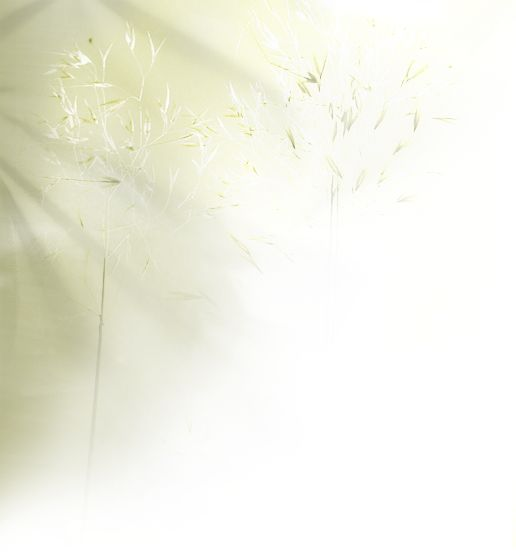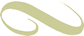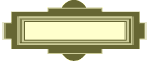Let it be - C
Intro: C    G      Am    F    C     G    F    C

C                   G                          Am              F
When I find myself in times of trouble, mother Mary comes to me,
C                          G                      F    C
speaking words of wisdom - - let it be.
C                      G                      Am                      F
And in my hour of darkness she is standing right in front of me,
C                        G                      F    C
speaking words of wisdom, - let it be.
Am        Em         F            C     C                        G                      F    C
Let it be, let it be, let it be, let it be. Whisper words of wisdom, - let it be.

C                        G                    Am              F
And when the broken hearted people living in the world agree,
C                      G                    F    C
there will be an answer - let it be.
C                             G                   Am                           F
For though they may be parted, there is still a chance that they will see,
C                        G                  F    C
there will be an answer - let it be.

Am        Em         F            C     C                      G                    F    C
% Let it be, let it be, let it be, let it be. There will be an answer, - let it be. %

% F    C    G-F    C %

C                        G                    Am                     F
And when the night is cloudy there is still a light that shines on me,
C                      G                  F    C
shine until tomorrow, - let it be.
C                      G                      Am               F
I wake up to the sound of music mother Mary comes to me,
C                              G                    F    C
speaking words of wisdom, - let it be.
Am        Em         F            C     C                          G                    F    C
Let it be, let it be, let it be, let it be. Whisper words of wisdom, - let it be.
Am        Em          F            C     C                      G                      F    C
Let it be, let it be, let it be, let it be. There will be an answer, - let it be.

% F    C    G-F    C %StartsideGitargrepLet it be mp3
Keyboardakkorder